# GED Math : Perimeter and Sides of Quadrilaterals

## Example Questions

← Previous 1 3 4 5

### Example Question #1 : Squares, Rectangles, And Parallelograms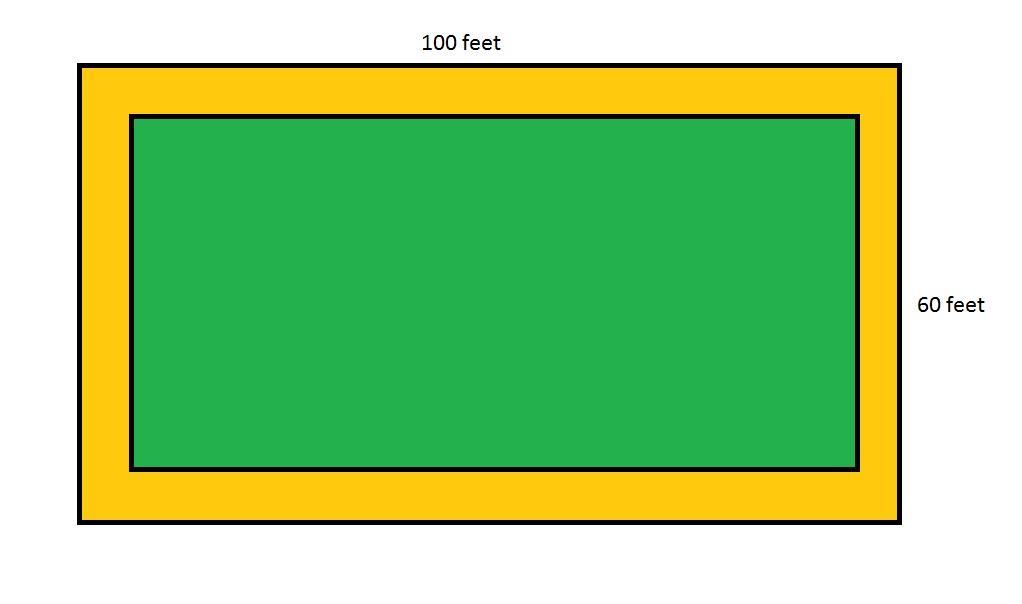Note:  Figure NOT drawn to scale

Refer to the above figure, which shows a rectangular garden (in green) surrounded by a dirt path (in brown) six feet wide throughout. What is the perimeter of the garden?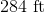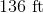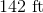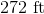Explanation:

The inner rectangle, which represents the garden, has length and width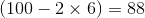feet and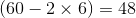feet, respectively, so its perimeter is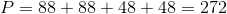feet.

### Example Question #2 : Squares, Rectangles, And Parallelograms

Which of the following can be the sidelengths of a rhombus?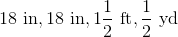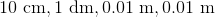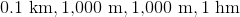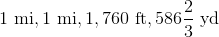Explanation:

The four sides of a rhombus have equal length, so we can eliminate three choices by demonstrating that at least two sidelengths are not equal.:

1,000 meters is, by definition, equal to 1 kilometer, not 0.1 kilometers. Therefore,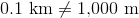and this choice is incorrect.:

1 mile is, by definition, equal to 5,280 feet, not 1,760 feet. Therefore,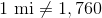and this choice is incorrect.By definition, 1 decimeter, not 0.1 decimeter, is equal to 1 meter. Therefore,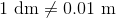and this choice is incorrect.:yard is equal to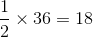inches and, also,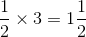feet. Therefore,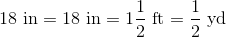All four sides have equal length so this is the rhombus. This is the correct choice.

### Example Question #1 : Squares, Rectangles, And Parallelograms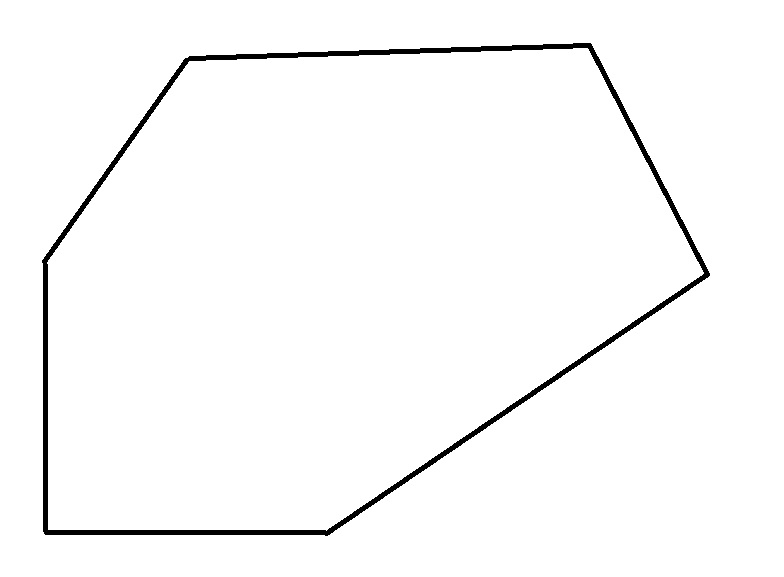Identify the above polygon.

Hexagon

Pentagon

Trapezoid

Rhombus

Hexagon

Explanation:

A polygon with six sides is called a hexagon.

### Example Question #1 : Squares, Rectangles, And Parallelograms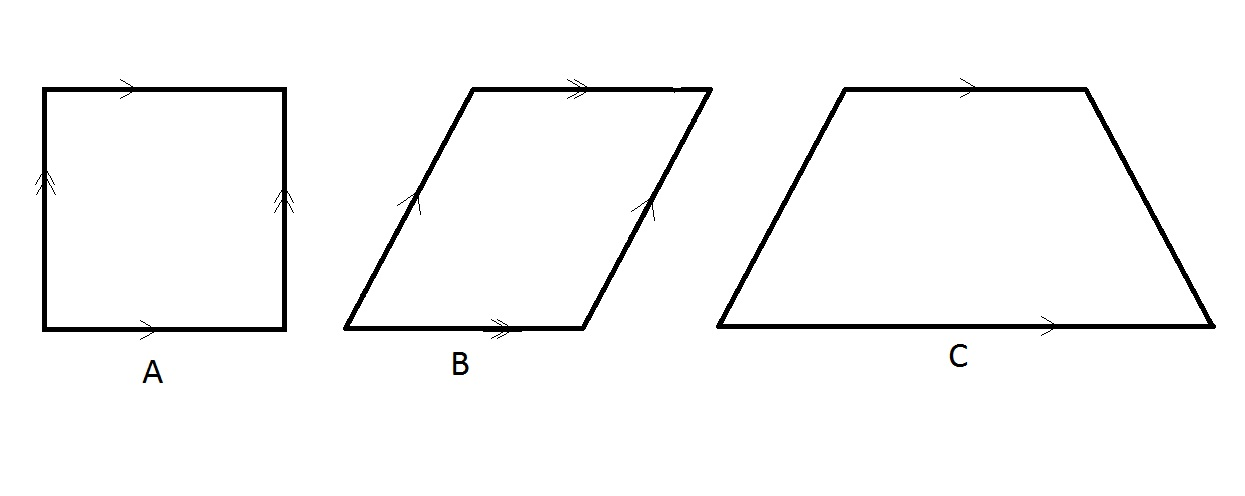Refer to the above three figures. All parallel sides are so indicated.

Which of the figures can be called a quadrilateral?

Figure C only

Figures A and B only

Figures B and C only

Figures A, B, and C

Figures A, B, and C

Explanation:

By definition, any polygon with four sides is called a quadrilateral. All three figures fit this description.

### Example Question #1 : Squares, Rectangles, And Parallelograms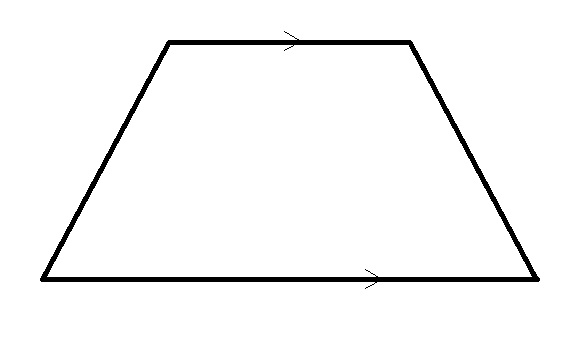Refer to the above diagram. Parallel sides are so indicated.

Identify the above polygon.

Pentagon

Trapezoid

Hexagon

Parallelogram

Trapezoid

Explanation:

A four-sided figure, or quadrilateral, with one pair of parallel sides and its other sides nonparallel is called a trapezoid.

### Example Question #1 : Squares, Rectangles, And Parallelograms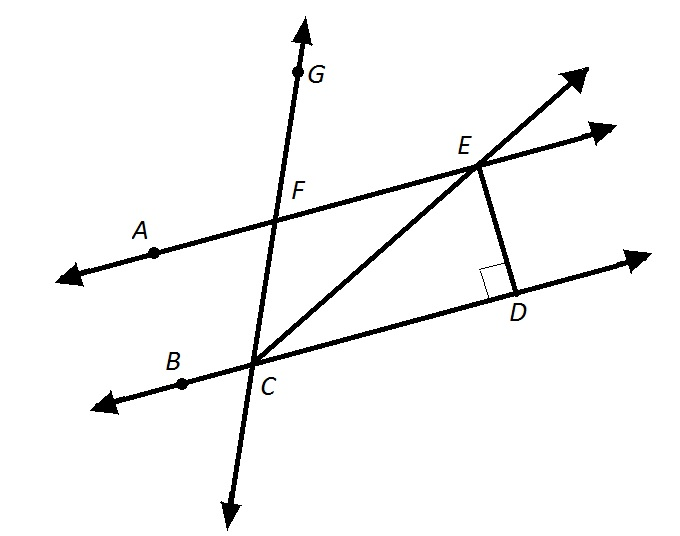Refer to the above figure. You are given that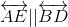and that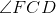is acute.

Which of the following words accurately describes Polygon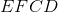?

Trapezoid

Pentagon

Parallelogram

Hexagon

Trapezoid

Explanation:

Polygonhas four sides and is therefore a quadrilateral., so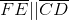. Also, sinceis acute and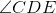is right,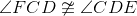, so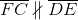The quadrilateral has one pair of parallel sides, and the other two sides are not parallel. Therefore, it is a trapezoid.

### Example Question #1 : Squares, Rectangles, And ParallelogramsRefer to the above three figures. All parallel sides are so indicated.

Which of the figures can be called a parallelogram?

Figure C only

Figure B only

Figures A, B, and C

Figures A and B only

Figures A and B only

Explanation:

A parallelogram, by definition, has two pairs of parallel sides. Figures A and B fit that criterion, but Figure C does not.

### Example Question #8 : Squares, Rectangles, And ParallelogramsNote:  Figure NOT drawn to scale.

Refer to the above figure, which shows a rectangular garden (in green) surrounded by a dirt path (in brown). The dirt path isfeet wide throughout. Which of the following polynomials gives the perimeter of the garden?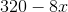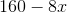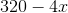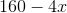Explanation:

The length of the garden isthan that of the entire lot, or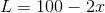.

The width of the garden isthan that of the entire lot, or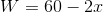.

The perimeter is twice the sum of the two: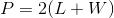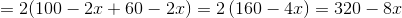### Example Question #9 : Squares, Rectangles, And Parallelograms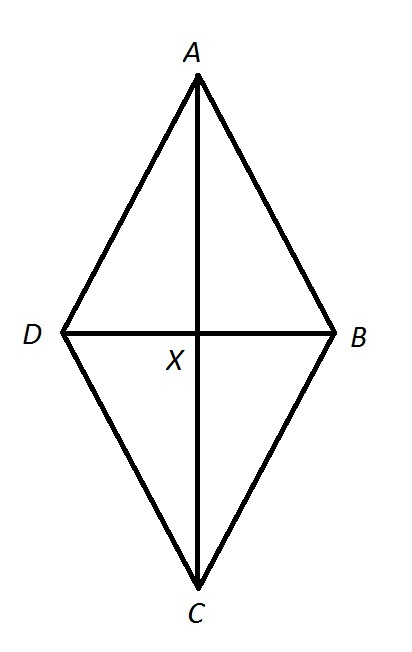Note: Figure NOT drawn to scale.

Quadrilateralis a rhombus. Calculate its perimeter if: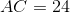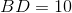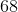Explanation:

The four sides of a rhombus are congruent. Also, the diagonals of a rhombus are perpendicular bisectors to each other, so the four triangles they form are right triangles. Therefore, the Pythagorean theorem can be used to determine the common sidelength of Quadrilateral.

We focus on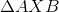. The diagonals of a rhombus, as is the case with any parallelogram, are each the other's bisector, so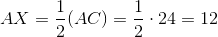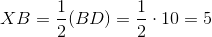By the Pythagorean Theorem,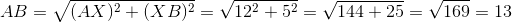13 is the common length of the four sides of Quadrilateral, so its perimeter is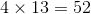.

### Example Question #10 : Squares, Rectangles, And Parallelograms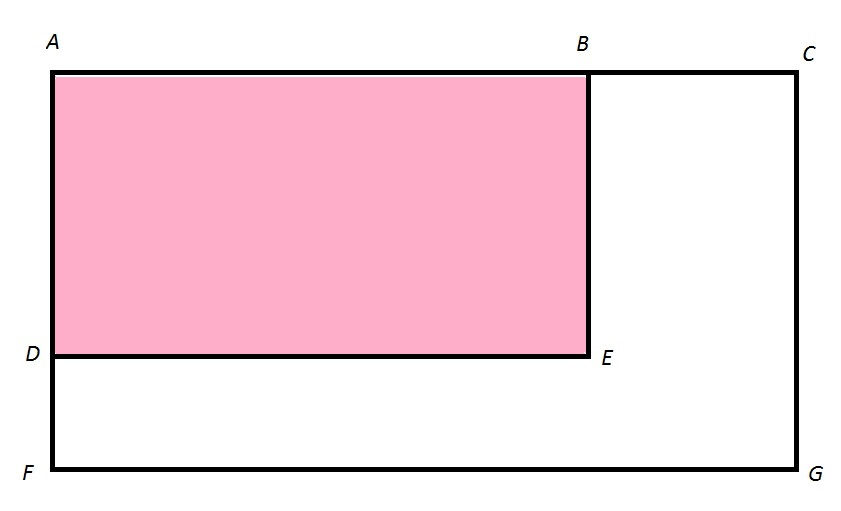Note: Figure NOT drawn to scale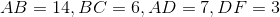Give the ratio of the perimeter of Rectangle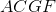to that of Rectangle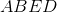.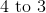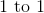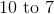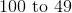Explanation:

The perimeter of Rectangleis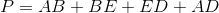.

Opposite sides of a rectangle are congruent, so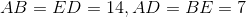and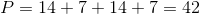.

The perimeter of Rectangleis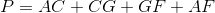.

Opposite sides of a rectangle are congruent, so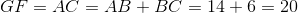,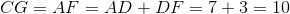,

and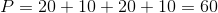.

The ratio of the perimeters is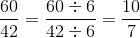- that is, 10 to 7.

← Previous 1 3 4 5

### All GED Math Resources